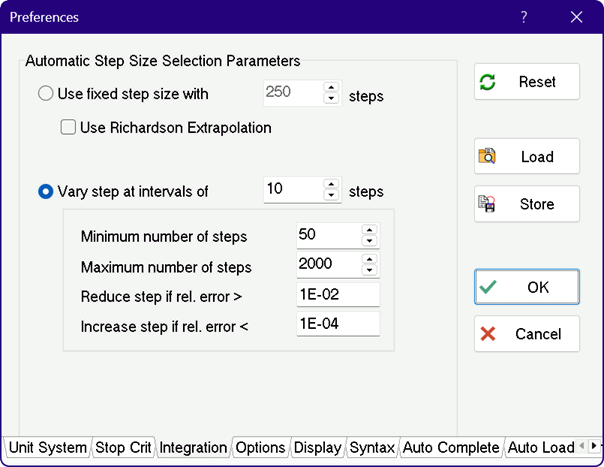Contents - Index

Integration Auto-Step Parameters

EES uses numerical integration to determine the value of an integral or to solve differential equations.  The equation-based Integral function can use either a fixed user-supplied step or an automatic step adjusted to meet some accuracy criteria.  If a fixed step is used, an option is provided to use Richardson Extrapolation to improve the numerical estimate.  The parameters in the Integration tab of the Preferences dialog, shown below, only affect the step size that EES automatically selects during numerical integration with the equation-based integral function.The two radio buttons control whether EES will use a fixed or a variable step.  Note that this option is used only when the step size is not specified in the equation-based Integral function. If the "Use fixed step size' button is selected, EES will use a fixed step equal to the interval divided by the number of steps indicated by the user.  If the "Use Richardson Extrapolation" box is checked, the Richardson extrapolation algorithm will be used with the fixed step size.  If "Vary step at intervals of" is selected, EES will check the numerical situation of the integration process every N steps where N is input by the user.  During this checking process, EES may decrease, increase, or maintain the current step size.  The minimum and maximum allowable steps and tolerances which control whether the step size is changed are input by the user.  The test that is done is as follows:

Every N steps EES will compare the value of the integral for that step with the value obtained using two half steps.  The difference between these two values is the truncation error.  The truncation error is normalized by dividing it by the value of the change in the integral for the two half-steps, assuming that it differs from zero.  If the normalized truncation error is greater than the value provided for 'Reduce step if rel. error >' the step size if reduced, assuming that it is greater than the minimum allowable step size.  If the normalized truncation error is less than the value provided for 'Increase step if rel. error <', the step is increased, provided that the increased step size does not exceed the maximum allowable step.  Otherwise, no change is made to the step size.  If an Integral Table is indicated with an unspecified output step size, the table will display the independent variable and specified variables at each integration step so the variation in the integration step size can be seen in the table. The maximum allowable step size is obtained by taking the integration time and dividing it by the Minimum Number of Steps.  The minimum allowable step size is obtained by taking the integration time and dividing it by the Maximum number of steps.

Note that there is an indirect relation between the Stop Criteria parameters and the integration truncation error parameters.  If the Stop Criteria parameters are set to allow convergence with errors larger than that used to reduce the step size, the calculation time and solution accuracy will both be adversely affected.

Also note that Richardson Extrapolation will not be used if the variable step size algorithm is employed.  If the step size is specified in the equation-based Integral command, e.g.,

Z=integral(X^2,X,0,1,0.1)

the specified step size (0.1 in the example above) is used overriding the setting made in this dialog.

________________________________________________________

Pressing the OK button will set the Integration tolerances for this session only.

Pressing the Store button will save the Integration tolerances along with all other information in the Preferences dialog an EES Preferences (*.prf) file.  EES will automatically load the EES.prf file when it is started if it is found in the same directory as the EES application.  An existing .prf file from any directory can be loaded with the Load button in the Preferences dialog or with a \$INCLUDE directive.  Note that the Integration tolerances are saved with other file information when the file is saved so that the Store button should be used only to store default values in a .prf file

The parameters for the automatic step size adjustment algorithm can also be changed with the \$IntegralAutoStep directive.